2015-12-21 17:11:35 baimafujinji 阅读数 12257
• ###### 机器学习案例实战第六课-PCA与Xgboost视频教学

购买课程后，可扫码进入学习群，获取唐宇迪老师答疑 用实例演示机器学习中的Xgboost算法和PCA原理。本视频课程首先讲解两个案例，基于文本分类的股价预测与PCA降维应用在鸢尾花数据集上。简介Xgboost算法原理，并安装xgboost实例演示如何进行操作。 会员卡优惠购买：http://edu.csdn.net/lecturer/1079

3151 人正在学习 去看看 唐宇迪

http://blog.csdn.net/baimafujinji/article/details/48467225

http://blog.csdn.net/baimafujinji/article/details/50372906

6.4.3 主成分变换的实现6.4.4 基于K-L变换的图像压缩pca变换 数字图像处理 相关内容

2019-07-22 20:15:17 Dandelion_2 阅读数 1235
• ###### 机器学习案例实战第六课-PCA与Xgboost视频教学

购买课程后，可扫码进入学习群，获取唐宇迪老师答疑 用实例演示机器学习中的Xgboost算法和PCA原理。本视频课程首先讲解两个案例，基于文本分类的股价预测与PCA降维应用在鸢尾花数据集上。简介Xgboost算法原理，并安装xgboost实例演示如何进行操作。 会员卡优惠购买：http://edu.csdn.net/lecturer/1079

3151 人正在学习 去看看 唐宇迪

PCA变换
PCA变换也称霍特林变换或K-L变换,是一种基于信息量的正交线性变换，该变换主要是采用线性投影的方法将数据投影到新的坐标空间中，从而使得新的成分按信息量分布,第一主成分包含的信息量最大，变换后各主成分分量彼此不相关，且随着主成分编号的增加该分量包含的信息量减小。PCA变换后图像的信息主要集中在前几个主成分分量中,在变换域中丢弃信息量小的主成分分量,将原始的海量高光谱数据变换为少量的几个成分，在降低数据维数的同时，最大限度地保持了原始数据的信息。PCA 广泛应用于图像压缩、图像增强、图像编码、随机噪声信号的去除及图像旋转等各种应用。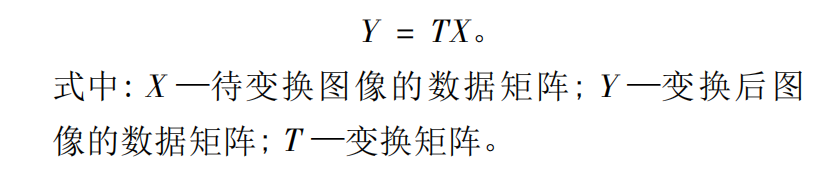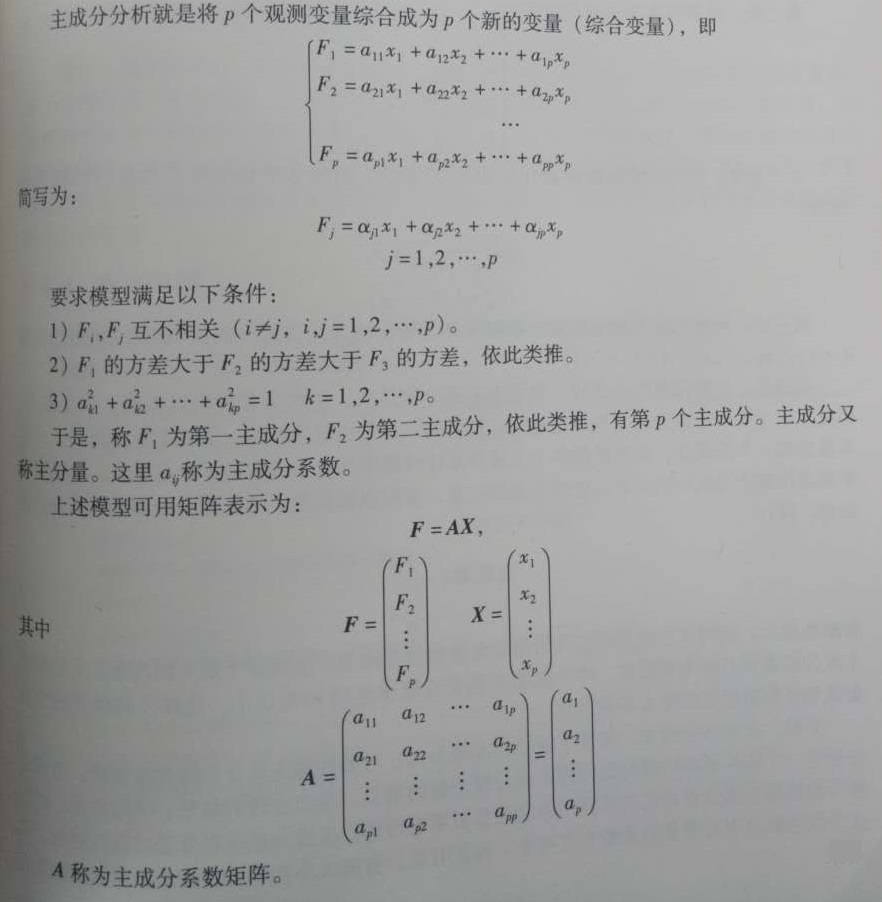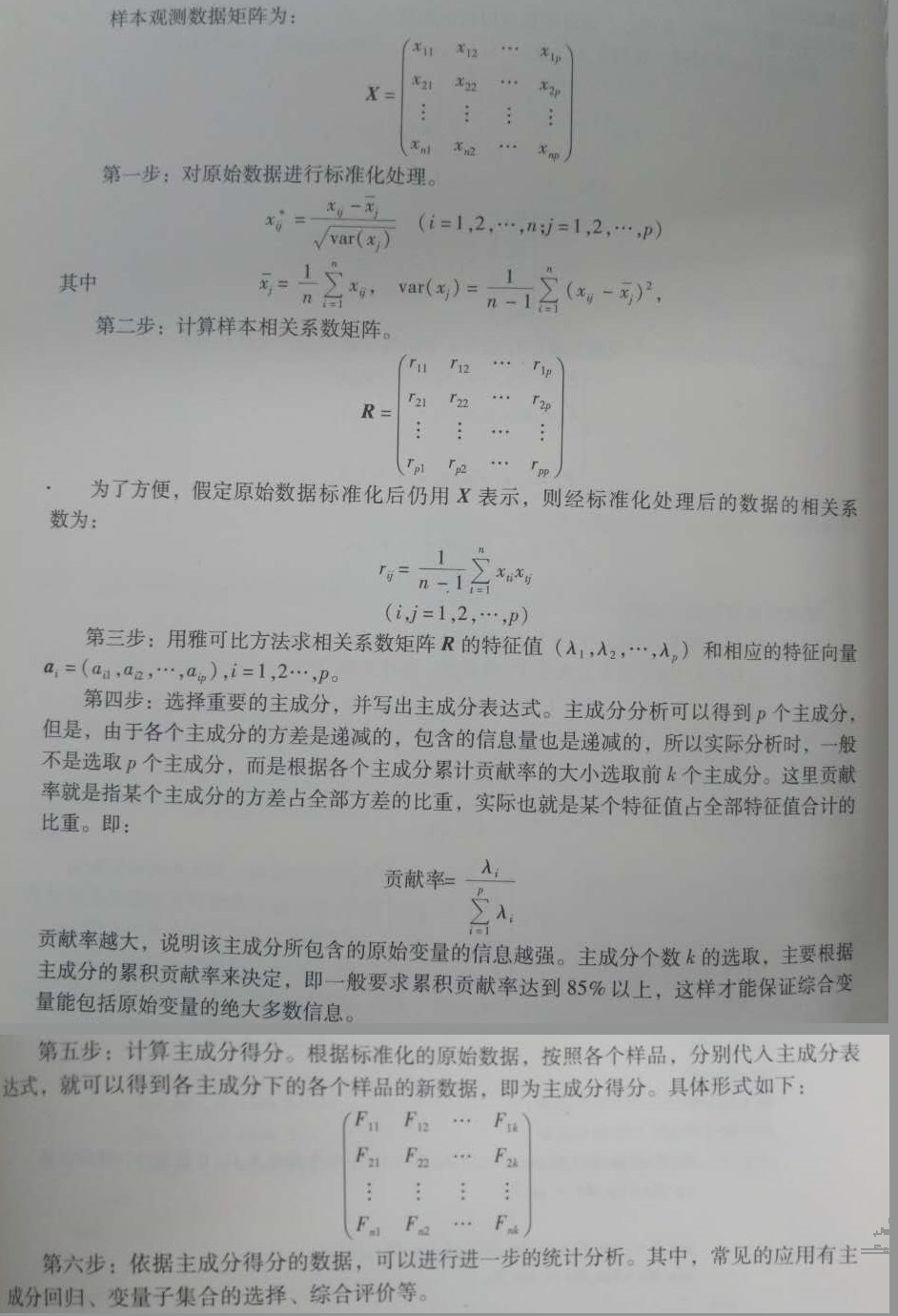PCA算法融合步骤如下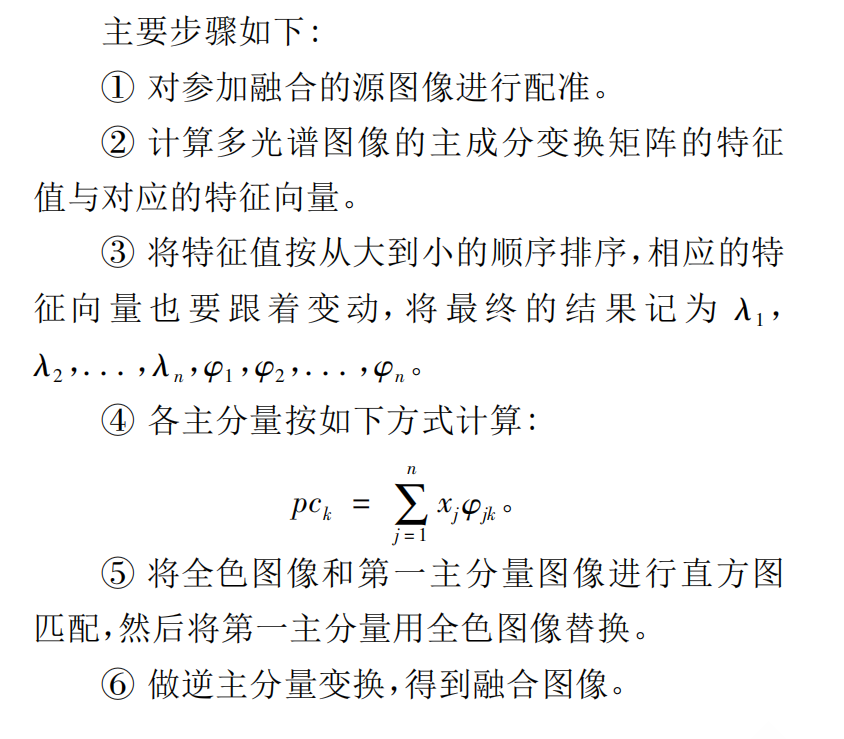PCA 变换融合方法的主要优点是:

``````% 基于PCA变换的图像融合方法

subplot(2,2,1);imshow(up);title('高分辨率图像');
subplot(2,2,2);imshow(low);title('低分辨率图像');

[up_R] = double(up(:,:,1));
[up_G] = double(up(:,:,2));
[up_B] = double(up(:,:,3));

[low_R] = double(low(:,:,1));
[low_G] = double(low(:,:,2));
[low_B] = double(low(:,:,3));

[M,N,color] = size(up);

up_Mx = 0;
low_Mx = 0;
for i = 1:M
for j = 1:N
up_S = [up_R(i,j),up_G(i,j),up_B(i,j)];         % 生成由RGB组成的三维列向量
up_Mx = up_Mx + up_S;                           % 叠加计算RGB各列向量的总和

low_S = [low_R(i,j),low_G(i,j),low_B(i,j)];
low_Mx = low_Mx + low_S;
end
end
up_Mx = up_Mx/(M * N);                                  % 计算三维列向量的平均值
low_Mx = low_Mx/(M * N);

up_Cx = 0;
low_Cx = 0;
for i = 1:M
for j = 1:N
up_S = [up_R(i,j),up_G(i,j),up_B(i,j)]';        % 矩阵转置
up_Cx = up_Cx + up_S * up_S';

low_S = [low_R(i,j),low_G(i,j),low_B(i,j)]';
low_Cx = low_Cx + low_S * low_S';
end
end
up_Cx = up_Cx/(M * N) - up_Mx * up_Mx';                 % 计算协方差矩阵
low_Cx = low_Cx/(M * N) - low_Mx * low_Mx';

[up_A,up_latent] = eigs(up_Cx);                         % 协方差矩阵的特征向量组成的矩阵
[low_A,low_latent] = eigs(low_Cx);                      % 即PCA变换的系数矩阵，特征值

for i = 1 : M
for j = 1 : N
up_X = [up_R(i,j),up_G(i,j),up_G(i,j)]';        % 生成由R，G， B组成的三维列
up_Y = up_A'*up_X;                              % 每个象素点进行PCA变换正变换
up_Y = up_Y';
up_R(i,j) = up_Y(1);                            % 高分辨率图片的第1主分量
up_G(i,j) = up_Y(2);                            % 高分辨率图片的第2主分量
up_B(i,j) = up_Y(3);                            % 高分辨率图片的第3主分量

low_X = [low_R(i,j),low_G(i,j),low_G(i,j)]';
low_Y = low_A'*low_X;
low_Y = low_Y';
low_R(i,j) = low_Y(1);                          % 低分辨率图片的第1主分量
low_G(i,j) = low_Y(2);                          % 低分辨率图片的第2主分量
low_B(i,j) = low_Y(3);                          % 低分辨率图片的第3主分量
end
end

for i = 1 : M
for j = 1 : N
up_Y = [up_R(i,j),up_G(i,j),up_B(i,j)]';         % 生成由R，G， B组成的三维列向量
up_X = up_A*up_Y;                                % 每个象素点进行PCA变换反变换
up_X = up_X';
up_r(i,j) = up_X(1);
up_g(i,j) = up_X(2);
up_b(i,j) = up_X(3);

low_Y = [up_R(i,j),low_G(i,j),low_B(i,j)]';
low_X = low_A*low_Y;
low_X = low_X';
low_r(i,j) = low_X(1);
low_g(i,j) = low_X(2);
low_b(i,j) = low_X(3);
end
end

RGB_up(:,:,1)=up_r;
RGB_up(:,:,2)=up_g;
RGB_up(:,:,3)=up_b;

RGB_low(:,:,1)=low_r;
RGB_low(:,:,2)=low_g;
RGB_low(:,:,3)=low_b;

subplot(2,2,3);imshow(uint8(RGB_up));title('高分辨率PCA变换图像');
subplot(2,2,4);imshow(uint8(RGB_low));title('低分辨率PCA变换图像');

``````

pca变换 数字图像处理 相关内容

2018-04-01 10:18:58 Chaolei3 阅读数 9145
• ###### 机器学习案例实战第六课-PCA与Xgboost视频教学

购买课程后，可扫码进入学习群，获取唐宇迪老师答疑 用实例演示机器学习中的Xgboost算法和PCA原理。本视频课程首先讲解两个案例，基于文本分类的股价预测与PCA降维应用在鸢尾花数据集上。简介Xgboost算法原理，并安装xgboost实例演示如何进行操作。 会员卡优惠购买：http://edu.csdn.net/lecturer/1079

3151 人正在学习 去看看 唐宇迪

https://blog.csdn.net/duanyule_cqu/article/details/54975867

*疑问：

# 提取并显示PCA 的各主成分

## 主代码

``````>> mul = imread('PCA/low.jpg');
>> extract_pca(mul);``````

## 实现 PCA的代码

``````function [vector ,value,tempMul] = my_pca(mul)
% % % % % % % % % % % % % % % % % % % % % % % % % % % % % %
% % % 该函数用来实现多光谱图像的PCA算法
% % %    param：
% % %          mul--输入的多光谱图像也可以是多通道的
% % %          value--特征值从大到小排列
% % %          vector--特征向量对应特征值排列，一列是一个特征向量
% % %          tempMul--mul的reshape成pixels*bands形式的2维矩阵
% % %
% % %  @author：chaolei
% % % % % % % % % % % % % % % % % % % % % % % % % % % % % %
mul=double(mul)/255;
[r ,c ,bands]=size(mul);
pixels = r*c;
% reshape成pixels*channel
mul = reshape(mul, [pixels,bands]);
tempMul = mul;
% 求各通道的均值
meanValue =  mean(mul,1);

% 数据去中心化
mul = mul - repmat(meanValue,[r*c,1]);
% 求协方差矩阵
correlation = (mul'*mul)/pixels;
%求特征向量与特征值
[vector ,value] = eig(correlation);
% 特征值和特征向量从大到小排序
vector = fliplr(vector);
value = fliplr(value);
value = flipud(value);

end``````

## 提取并显示PCA的各主成分

``````function extract_pca(mul)
% % % % % % % % % % % % % % % % % % % % % % % % % % % % % %
% % % 该函数用来抽取并显示pca的各个主成分
% % %   param：
% % %       mul--输入图像是多光谱图像或者高光谱图像
% % %  @author：chaolei
% % % % % % % % % % % % % % % % % % % % % % % % % % % % % %
[r,c,bands]=size(mul);
[vector,~,tempMul]= my_pca(mul);
% Y=AX（X中列为样本，若X行为样本，则Y =XA）
% PCA正变换
PC = tempMul*vector;
% 提取多光谱图像的各个主成分
for i = 1:bands
outPic = PC(:,i);
min_value = min(outPic);
max_value = max(outPic);
outPic = reshape(outPic,[r,c]);
figure;
str = sprintf('%s%d%s','第',i,'主成分');
imshow(outPic,[min_value,max_value]);title(str);
%     filename = sprintf('%d%s',i,'.jpg');
%     imwrite(outPic,filename);
end
end``````

## 结果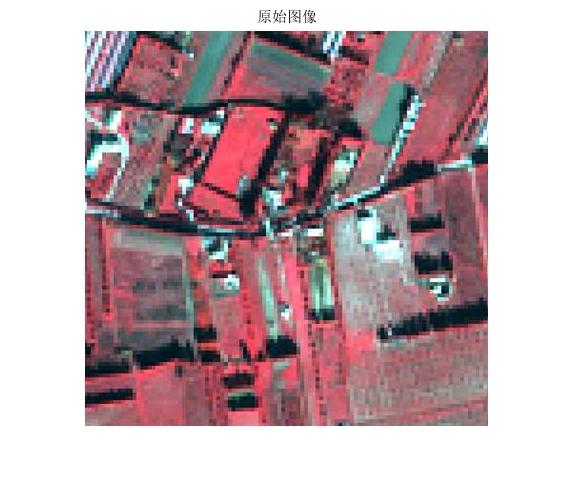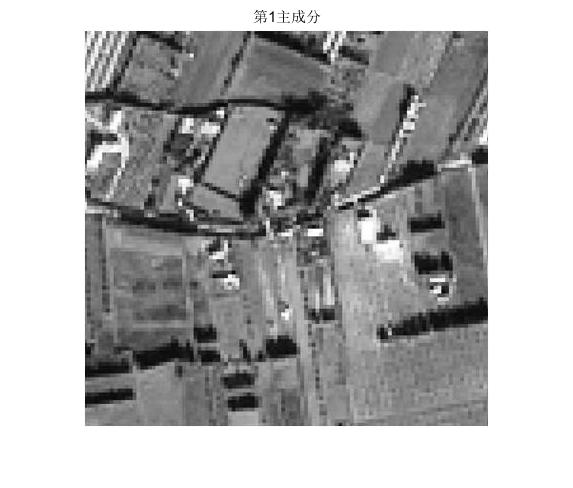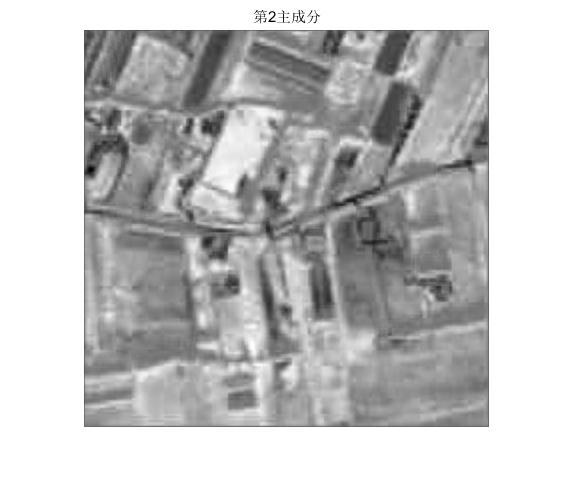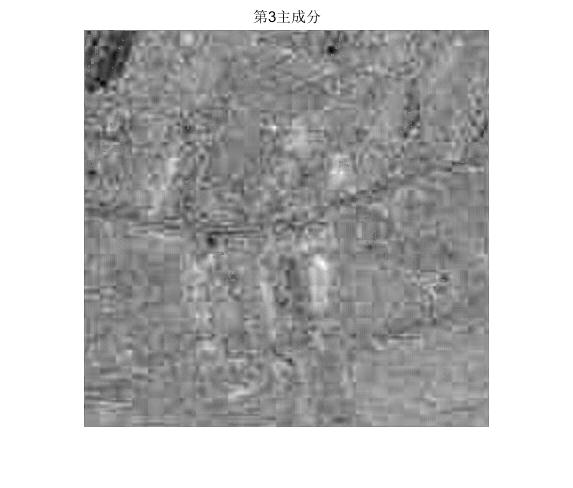# 实现PCA进行压缩

``````function  pca_comp_show(mul,n)
% % % % % % % % % % % % % % % % % % % % % % % % % % % % % % % %
% % % 该函数用来显示经过PCA压缩后的图像
% % %  param：
% % %     mul--输入的多光谱或者高光谱图像
% % %     n--指定用多少个主成分
% % % @author：chaolei
% % % % % % % % % % % % % % % % % % % % % % % % % % % % % % % %
[vector ,value,tempMul] = my_pca(mul);
% 使用其中最重要的n个主成分,并还原到原图像大小
% PCA逆变换
% 原始数据reshape*特征向量矩阵*特征向量矩阵'
re = tempMul*vector(:,1:n)*vector(:,1:n)';
[r,c,bands] =size(mul);
comp = reshape(re,[r,c,bands]);

str =sprintf('%d%s',n,'个主成分');
figure;imshow(comp);title(str);
end``````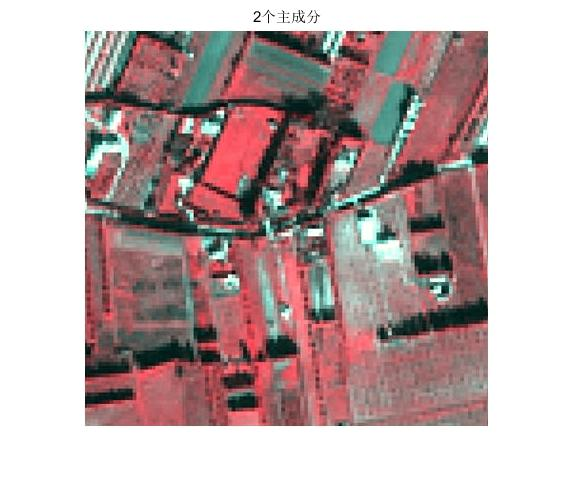pca变换 数字图像处理 相关内容

2015-12-21 16:47:14 baimafujinji 阅读数 13928
• ###### 机器学习案例实战第六课-PCA与Xgboost视频教学

购买课程后，可扫码进入学习群，获取唐宇迪老师答疑 用实例演示机器学习中的Xgboost算法和PCA原理。本视频课程首先讲解两个案例，基于文本分类的股价预测与PCA降维应用在鸢尾花数据集上。简介Xgboost算法原理，并安装xgboost实例演示如何进行操作。 会员卡优惠购买：http://edu.csdn.net/lecturer/1079

3151 人正在学习 去看看 唐宇迪

http://blog.csdn.net/baimafujinji/article/details/48467225

6.4.2 主成分变换的推导pca变换 数字图像处理 相关内容

2019-11-01 11:23:03 weixin_43262648 阅读数 110
• ###### 机器学习案例实战第六课-PCA与Xgboost视频教学

购买课程后，可扫码进入学习群，获取唐宇迪老师答疑 用实例演示机器学习中的Xgboost算法和PCA原理。本视频课程首先讲解两个案例，基于文本分类的股价预测与PCA降维应用在鸢尾花数据集上。简介Xgboost算法原理，并安装xgboost实例演示如何进行操作。 会员卡优惠购买：http://edu.csdn.net/lecturer/1079

3151 人正在学习 去看看 唐宇迪

# 数字图像处理——影像融合

## 1. Brovey变换

``````for k = 1:bands1
for i = 1:lines2
for j = 1:samples2
Image3(i,j,k) = Image2(i,j,k)*Image1(i,j,k)/sum(Image1(i,j,:));
end
end
end
figure(1)
subplot(1,3,1);
imshow(uint8(Image1));
title('高光谱影像');
subplot(1,3,2);
imshow(uint8(Image2));
title('全色影像');
subplot(1,3,3);
imshow(uint8(Image3));
title('Brovey变换融合后的影像');``````## 2.乘积变换

``````for k =1:bands1
for i = 1:lines2
for j = 1:samples2
Image3(i,j,k) = Image1(i,j,k)*Image2(i,j,k);
end
end
end
Image3 = round((Image3 - min(Image3))*255./(max(Image3) - min(Image3)));
figure(2)
subplot(1,3,1);
imshow(uint8(Image1));
title('高光谱影像');
subplot(1,3,2);
imshow(uint8(Image2));
title('全色影像');
subplot(1,3,3);
imshow(uint8(Image3));
title('乘积变换融合后的影像');``````## 3.PCA变换

``````AY = PCA(Image1);
A = cell2mat(AY(1));
Y = round(cell2mat(AY(2)));
YY = matching(Image2(:,:,1),reshape(Y(1,:),[lines1,samples1]));
Y(1,:) = reshape(YY,[1,lines2*samples2]);
X = double(uint8(inv(A)* Y));
for i = 1:bands1
Image3(:,:,i) = reshape(X(i,:),[lines1,samples1]);
end
figure(3);
subplot(1,3,1);
imshow(uint8(Image1));
title('高光谱影像');
subplot(1,3,2);
imshow(uint8(Image2));
title('全色影像');
subplot(1,3,3);
imshow(uint8(Image3));
title('PCA融合后的影像');``````## 4.HSI变换

``````HSI = RGB2HSI(Image1);
max = max(max(HSI(:,:,3)));
min = min(min(HSI(:,:,3)));
HSI(:,:,3) = round(HSI(:,:,3));
I = matching(Image2(:,:,1),HSI(:,:,3));
% I = Image2(:,:,1);
HSI(:,:,3) = I;
Image3 =HSI2RGB(HSI);
figure(4)
subplot(1,3,1);
imshow(uint8(Image1));
title('高光谱影像');
subplot(1,3,2);
imshow(uint8(Image2));
title('全色影像');
subplot(1,3,3);
imshow(uint8(Image3));
title('HSI变换融合后的影像');``````pca变换 数字图像处理 相关内容